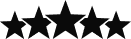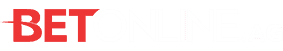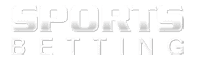Top 5 CS:GO Betting Sites
1VISIT NOW
2VISIT NOW
3VISIT NOW
4VISIT NOW
5VISIT NOW

If you approach CSGO betting in the right way, you can make some money from it. However, before you even try to do this, you will need to make sure that you have some understanding of how betting odds work. Making an error and betting on the wrong odds can be costly, so it’s important you understand them inside and out. The idea behind betting odds is simple in that they imply the chance of how likely something is going to happen. For example, the decimal odds of 2.00 suggest something has a 50% chance of happening. At these odds, you will double your money if your bet wins.

If you want to drill down into the most basic level of betting odds, then you need to look at probability. This is how probable something is to happen. For any given event, there are a number of possible outcomes, even something as a simple rolling a dice, which has 6 different faces, and therefore 6 different possible outcomes. This means that rolling a “one” has a probability of 16.67%.

## Fractional Odds

Betting odds are most commonly displayed as a fraction on betting sites rather than a percentage. For example, you might see “9/1”. A calculation is used in order to work these odds out. For the purpose of explanation we’ll show 9/1 as A/B. This calculation is:

• Probability (%) = B / (A+B)

So, if you had odds of 9/1 then the calculation would be 1 / (9+1) = 0.10. This means there is a 10% chance that an event will happen. Not all odds are presented as something / 1. Therefore, if you see odds of 1/4 then the calculation tells us that there is an 80% chance of that event happening.

## Fractional Odds Winnings

It isn’t only probability that these odds are used to calculate, but they are also used to show how much you are likely to win if the event does happen. This calculation is a bit simpler, all you need to do is present the odds as A/B and for every B that you bet, you will win A, plus your stake.

## Decimal Odds

Although fractional odds are the more traditional approach, more CSGO betting sites are starting to use decimal odds. Although, these are still not quite as common, they are more likely to be seen on betting exchanges. The calculation for decimal odds is:

• Winnings = (odds * stake) – stake

An example of this is:

• 9.0 odds (9.0 * £10 stake) -£10 stake = £80 winnings

Decimal odds can be easier to understand, and many betting sites give you the opportunity to only present your odds in this way. It really is down to personal preference as to which odds format you choose. Just make sure you understand how much you are risking in relation to how much it’s possible you can win. Hopefully now you understand betting odds and their various formats. Good luck with your CSGO bets!